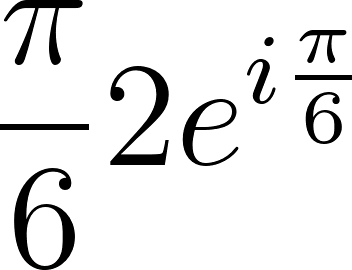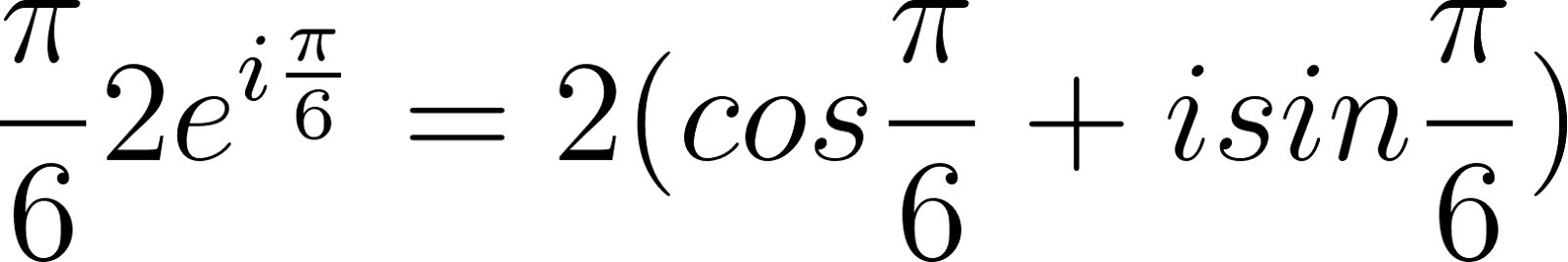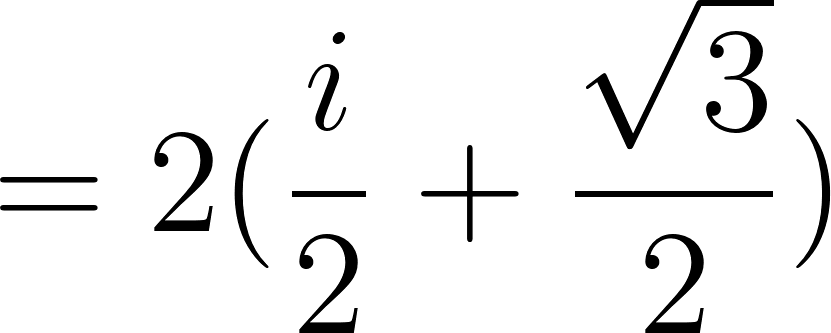Modern Algebra: An Introduction - 6 Edition - Chapter 33 - Problem 33.1
Register Now

Join StudySoup

Get Full Access to Modern Algebra: An Introduction - 6 Edition - Chapter 33 - Problem 33.19780470384435

# Write each of the following complex numbers in the form a + bi. The number with absolute

Modern Algebra: An Introduction | 6th Edition

Problem 33.1

Write each of the following complex numbers in the form a + bi. The number with absolute value 2 and argument rr /6.

Accepted Solution
Step-by-Step Solution:

Step 1 of 2

The modulus of a complex number is 2 and the argument is. From De movies theorem we get###### Chapter 33, Problem 33.1 is Solved

Step 2 of 2

Unlock Textbook Solution